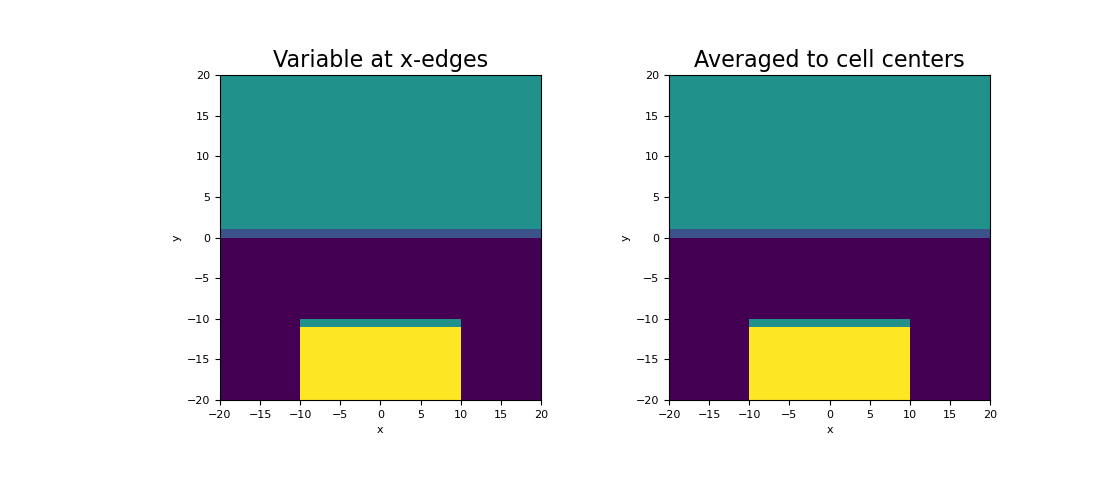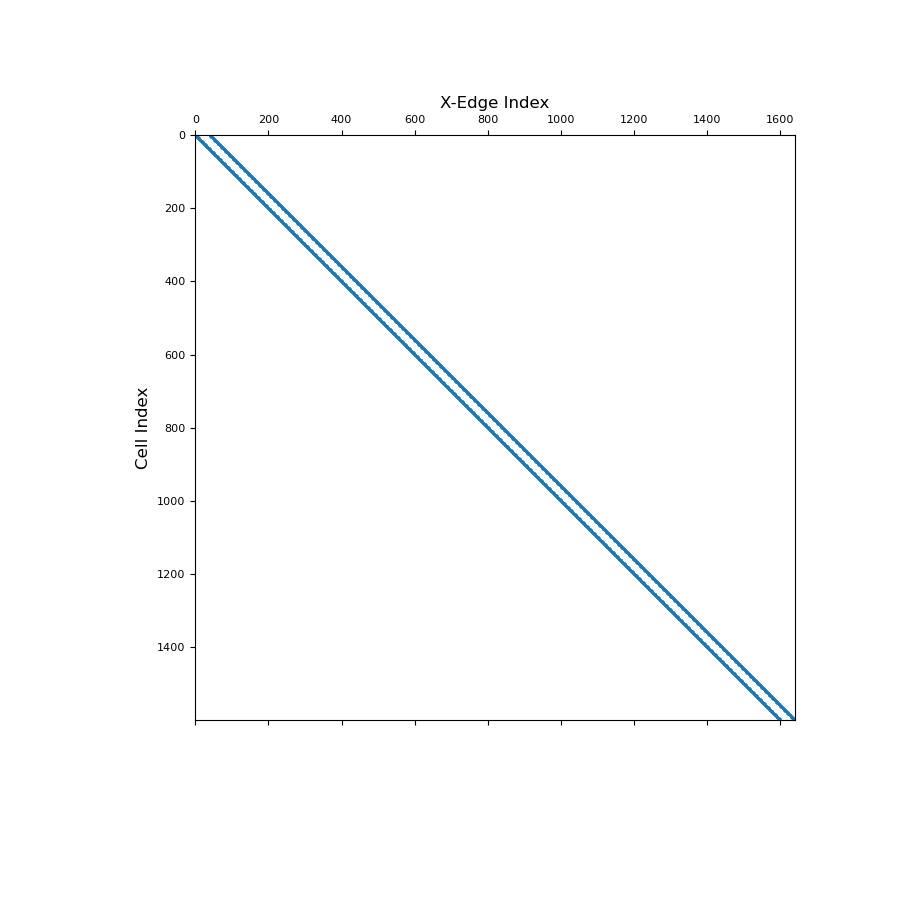# discretize.CylindricalMesh.average_edge_x_to_cell¶

property CylindricalMesh.average_edge_x_to_cell

Averaging operator from x-edges to cell centers (scalar quantities).

This property constructs a 2nd order averaging operator that maps scalar quantities from x-edges to cell centers. This averaging operator is used when a discrete scalar quantity defined on x-edges must be projected to cell centers. Once constructed, the operator is stored permanently as a property of the mesh. See notes.

Returns
(n_cells, n_edges_x) scipy.sparse.csr_matrix

The scalar averaging operator from x-edges to cell centers

Notes

Let $$\boldsymbol{\phi_x}$$ be a discrete scalar quantity that lives on x-edges. average_edge_x_to_cell constructs a discrete linear operator $$\mathbf{A_{xc}}$$ that projects $$\boldsymbol{\phi_x}$$ to cell centers, i.e.:

$\boldsymbol{\phi_c} = \mathbf{A_{xc}} \, \boldsymbol{\phi_x}$

where $$\boldsymbol{\phi_c}$$ approximates the value of the scalar quantity at cell centers. For each cell, we are simply averaging the values defined on its x-edges. The operation is implemented as a matrix vector product, i.e.:

phi_c = Axc @ phi_x


Examples

Here we compute the values of a scalar function on the x-edges. We then create an averaging operator to approximate the function at cell centers. We choose to define a scalar function that is strongly discontinuous in some places to demonstrate how the averaging operator will smooth out discontinuities.

We start by importing the necessary packages and defining a mesh.

>>> from discretize import TensorMesh
>>> import numpy as np
>>> import matplotlib.pyplot as plt
>>> h = np.ones(40)
>>> mesh = TensorMesh([h, h], x0="CC")


Then we create a scalar variable on x-edges,

>>> phi_x = np.zeros(mesh.nEx)
>>> xy = mesh.edges_x
>>> phi_x[(xy[:, 1] > 0)] = 25.0
>>> phi_x[(xy[:, 1] < -10.0) & (xy[:, 0] > -10.0) & (xy[:, 0] < 10.0)] = 50.0


Next, we construct the averaging operator and apply it to the discrete scalar quantity to approximate the value at cell centers.

>>> Axc = mesh.average_edge_x_to_cell
>>> phi_c = Axc @ phi_x


And plot the results,

Expand to show scripting for plot
>>> fig = plt.figure(figsize=(11, 5))
>>> v = np.r_[phi_x, np.zeros(mesh.nEy)]  # create vector for plotting function
>>> mesh.plot_image(v, ax=ax1, v_type="Ex")
>>> ax1.set_title("Variable at x-edges", fontsize=16)
>>> mesh.plot_image(phi_c, ax=ax2, v_type="CC")
>>> ax2.set_title("Averaged to cell centers", fontsize=16)
>>> plt.show()Below, we show a spy plot illustrating the sparsity and mapping of the operator

Expand to show scripting for plot
>>> fig = plt.figure(figsize=(9, 9))